Documentation

## How to Choose Knots

This example shows how to select and optimize knots using the `optknt` and `newknt` commands from Curve Fitting Toolbox™.

### Sample Data

Here are some sample data, much used for testing spline approximation with variable knots, the so-called Titanium Heat Data. They record some property of titanium measured as a function of temperature.

```[xx,yy] = titanium; plot(xx,yy,'x'); axis([500 1100 .55 2.25]); title('The Titanium Heat Data'); hold on```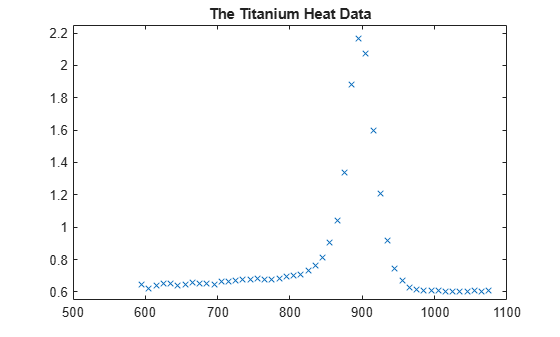Notice the rather sharp peak. We'll use these data to illustrate some methods for knot selection.

First, we pick a few data points from these somewhat rough data. We will interpolate using this subset, then compare results to the full dataset.

```pick = [1 5 11 21 27 29 31 33 35 40 45 49]; tau = xx(pick); y = yy(pick); plot(tau,y,'ro'); legend({'Full Dataset' 'Subsampled Data'}, 'location','NW');```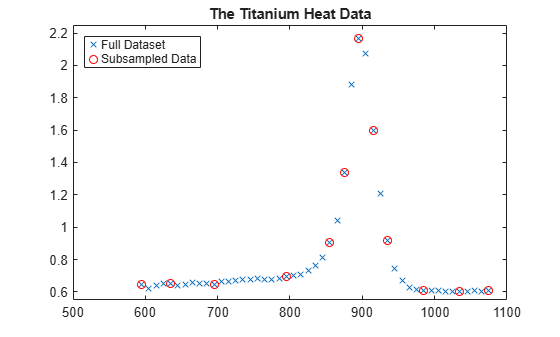### General Considerations

A spline of order `k` with `n+k` knots has `n` degrees of freedom. Since we have 12 data sites, `tau(1) < ... < tau(12)`, a fit with a cubic spline, i.e., a fourth order spline, requires a knot sequence `t` of length 12+4.

Moreover, the knot sequence `t` must satisfy the Schoenberg-Whitney conditions, i.e., must be such that the i-th data site lies in the support of the i-th B-spline, i.e.,

` t(i) < tau(i) < t(i+k) for all i,`

with equality allowed only in case of a knot of multiplicity `k`.

One way to choose a knot sequence satisfying all these conditions is as the optimal knots, of Gaffney/Powell and Micchelli/Rivlin/Winograd.

### Optimal Knots

In optimal spline interpolation, to values at sites

` tau(1), ..., tau(n)`

say, the knots are chosen so as to minimize the constant in a standard error formula. Specifically, the first and the last data site are chosen as k-fold knots. The remaining `n-k` knots are supplied by `optknt`.

Here is the beginning of the help from `optknt`:

OPTKNT Optimal knot distribution.

`OPTKNT(TAU,K) returns an `optimal' knot sequence for`

`interpolation at data sites TAU(1), ..., TAU(n) by splines of`

`order K. TAU must be an increasing sequence, but this is not`

`checked.`

`OPTKNT(TAU,K,MAXITER) specifies the number MAXITER of iterations`

`to be tried, the default being 10.`

`The interior knots of this knot sequence are the n-K`

`sign-changes in any absolutely constant function h ~= 0 that`

`satisfies`

` integral{ f(x)h(x) : TAU(1) < x < TAU(n) } = 0`

`for all splines f of order K with knot sequence TAU.`

### Trying OPTKNT

We try using `optknt` for interpolation on our example, interpolating by cubic splines to data

` (tau(i), y(i)), for i = 1, ..., n.`

```k = 4; osp = spapi( optknt(tau,k), tau,y); fnplt(osp,'r'); hl = legend({'Full Dataset' 'Subsampled Data' ... 'Cubic Spline Interpolant Using Optimal knots'}, ... 'location','NW'); hl.Position = hl.Position-[.14,0,0,0];```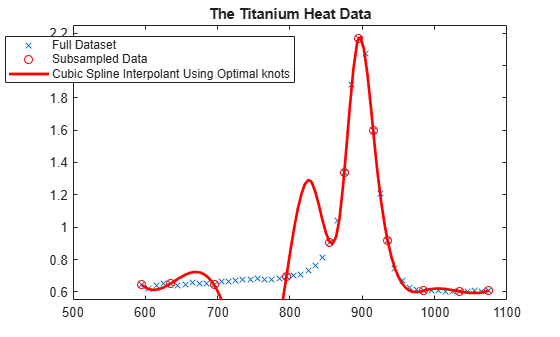This is a bit disconcerting!

Here, marked by stars, are also the interior optimal knots:

```xi = fnbrk(osp,'knots'); xi([1:k end+1-(1:k)]) = []; plot(xi,repmat(1.4, size(xi)),'*'); hl = legend({'Full Dataset' 'Subsampled Data' ... 'Cubic Spline Interpolant Using Optimal knots' ... 'Optimal Knots'}, 'location','NW'); hl.Position = hl.Position-[.14,0,0,0];```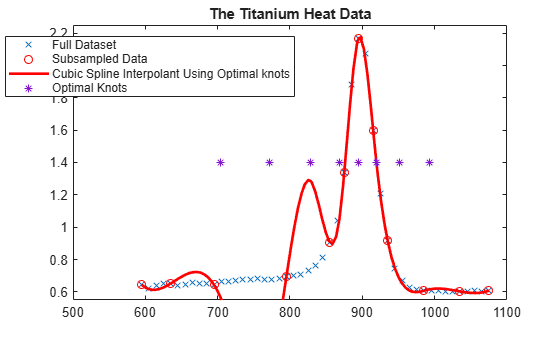### What Happened?

The knot choice for optimal interpolation is designed to make the maximum over all functions `f` of the ratio

` norm(f - If) / norm(D^k f)`

as small as possible, where the numerator is the norm of the interpolation error, `f - If`, and the denominator is the norm of the `k`-th derivative of the interpolant, `D^k f`. Since our data imply that `D^k f` is rather large, the interpolation error near the flat part of the data is of acceptable size for such an `optimal' scheme.

Actually, for these data, the ordinary cubic spline interpolant provided by `csapi` does quite well:

```cs = csapi(tau,y); fnplt(cs,'g',2); hl = legend({'Full Dataset' 'Subsampled Data' ... 'Cubic Spline Interpolant Using Optimal knots' ... 'Optimal Knots' 'Cubic Spline Interpolant Using CSAPI'}, ... 'location','NW'); hl.Position = hl.Position-[.14,0,0,0]; hold off```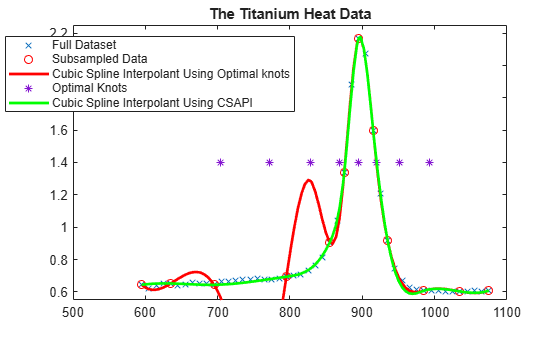### Knot Choice for Least Squares Approximation

Knots must be selected when doing least-squares approximation by splines. One approach is to use equally-spaced knots to begin with, then use `newknt` with the approximation obtained for a better knot distribution.

The next sections illustrate these steps with the full titanium heat data set.

### Least Squares Approximation with Uniform Knot Sequence

```unif = linspace(xx(1), xx(end), 2+fix(length(xx)/4)); sp = spap2(augknt(unif, k), k, xx, yy); plot(xx,yy,'x'); hold on fnplt(sp,'r'); axis([500 1100 .55 2.25]); title('The Titanium Heat Data'); hl = legend({'Full Dataset' ... 'Least Squares Cubic Spline Using Uniform Knots'}, ... 'location','NW'); hl.Position = hl.Position-[.14,0,0,0];```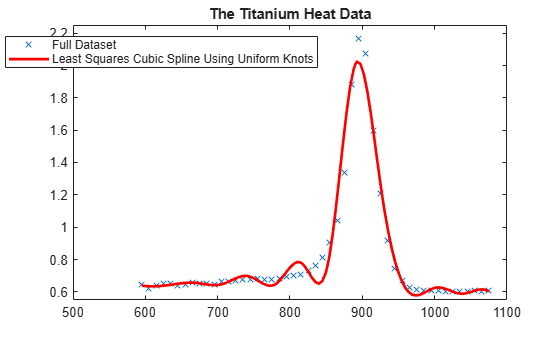This is not at all satisfactory. So we use `newknt` for a spline approximation of the same order and with the same number of polynomial pieces, but the breaks better distributed.

### Using NEWKNT to Improve the Knot Distribution

```spgood = spap2(newknt(sp), k, xx,yy); fnplt(spgood,'g',1.5); hl = legend({'Full Dataset' ... 'Least Squares Cubic Spline Using Uniform Knots' ... 'Least Squares Cubic Spline Using NEWKNT'}, ... 'location','NW'); hl.Position = hl.Position-[.14,0,0,0]; hold off```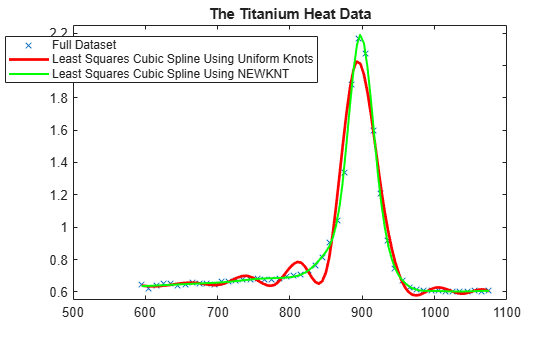This is quite good. Incidentally, even one interior knot fewer would not have sufficed in this case.